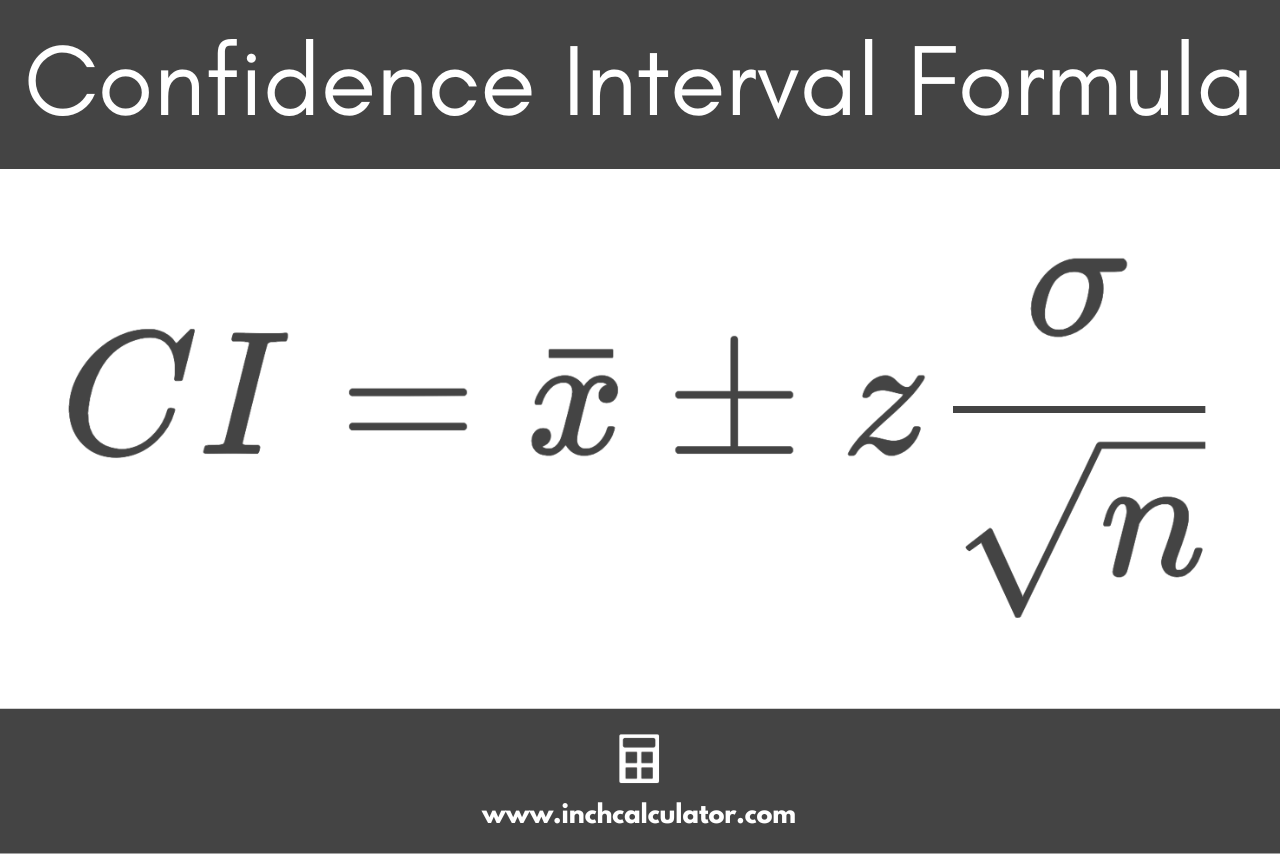# Confidence Interval Calculator

Enter the confidence level, sample size, sample mean, and standard deviation to find the confidence interval using the calculator below.

## Results:

### Steps to Solve

#### Confidence Interval Formula

CI = x̄ ± z × σ / √n

z-Score = ?

SE = σ / √n

MOE = z × SE

#### Step Four: Find the Confidence Interval

CI = x̄ ± MOE

Learn how we calculated this below

## How to Find the Confidence Interval

In statistics, a confidence interval is a range of values from a sample that are likely to contain an unknown population parameter value.

Because surveys are often done on a sample of a larger population, there will inevitably be some amount of error or difference between the sample and the full population, which is known as the margin of error.

The confidence interval is used to measure the uncertainty in a sample variable. It’s essentially the range of the sample data that is expected to reflect a variable within the full population.For instance, if you have a sample and know the mean of a variable in the sample, you can make an estimation with some level of certainty that the mean of the population will fall within a range. The confidence interval is that range.

Because nothing is ever certain, statisticians also use a confidence level, which is the probability that a variable in the full population falls within the confidence interval range.

So if you’re using a 95% confidence level, then you might say that there is a 95% chance that the population mean will fall within a confidence interval range. The higher the confidence level, the wider the confidence interval will be, and vice versa.

The confidence interval is a range between a lower and upper bound. These bounds are equal to the sample mean minus the margin of error and the sample mean plus the margin of error, respectively.

You can use our margin of error calculator to find the margin of error for your sample.

A smaller confidence interval means that the range between the upper and lower bounds is narrower, and a larger confidence interval means that the range is wider. The narrower this range is, the more precise the estimate will be.

### Confidence Interval Formula

To find the confidence interval, you can use the following formula:

CI = x̄ ± z × σ / √n

Thus, the confidence interval is equal to the sample mean plus or minus the z-score for the confidence level z times the population standard deviation σ divided by the square root of the sample size n. Ideally, the standard deviation of the population would be used with this formula, but if you don’t know it, then the sample standard deviation can be used.You can use this formula to calculate the confidence interval in four easy steps.

### Step One: Find the Z-Score

You can find the critical value for your desired confidence level for a standard normal distribution (aka z-score) using the z-score table below. Learn more about z-values on our z-score calculator.

### Step Two: Find the Standard Error

The standard error is equal to the population standard deviation divided by the square root of the sample size. That’s encapsulated in this portion of the formula above:

SE = σ / √n

### Step Three: Find the Margin of Error

You can calculate the margin of error by multiplying the standard error by the z-score from the steps above.

MOE = z × SE

### Step Four: Find the Confidence Interval

The final step to calculate the confidence interval is to add and subtract the margin of error from the sample mean to find the upper and lower bounds.

upper bound = x̄ + MOE
lower bound = x̄ – MOE

Thus, you could say that the confidence interval is equal to the sample mean plus or minus the margin of error.

CI = x̄ ± MOE

### Example: Calculate a 95% Confidence Interval

For example, let’s find the confidence interval for a sample with a mean of 14, a standard deviation of 2.5, and a size of 1500. Using a confidence level of 95%, we can use a z-score of 1.96 (see the table below).

Start by assigning values to the variables needed for the formula.

CI = 14 ± 1.96 × 2.5 / √1500
CI = 14 ± 1.96 × 2.5 / 38.73
CI = 14 ± 1.96 × 0.065
CI = 14 ± 0.1274

Thus, the confidence interval for this sample is equal to 14 plus or minus 0.1274.

You can find the lower and upper bounds to fully define the range for the confidence interval.

lower bound = 14 – 0.1274 = 13.8726
upper bound = 14 + 0.1274 = 14.1274

So, the confidence interval for this sample is between 13.8726 and 14.1274.

### Z-Scores

The table below shows the z-values for various confidence levels.

Table showing the z-scores for various confidence levels.
Confidence Level Z-Score
70% 1.04
75% 1.15
80% 1.28
85% 1.44
90% 1.645
91% 1.7
92% 1.75
93% 1.81
94% 1.88
95% 1.96
96% 2.05
97% 2.17
98% 2.33
99% 2.576
99.9% 3.29